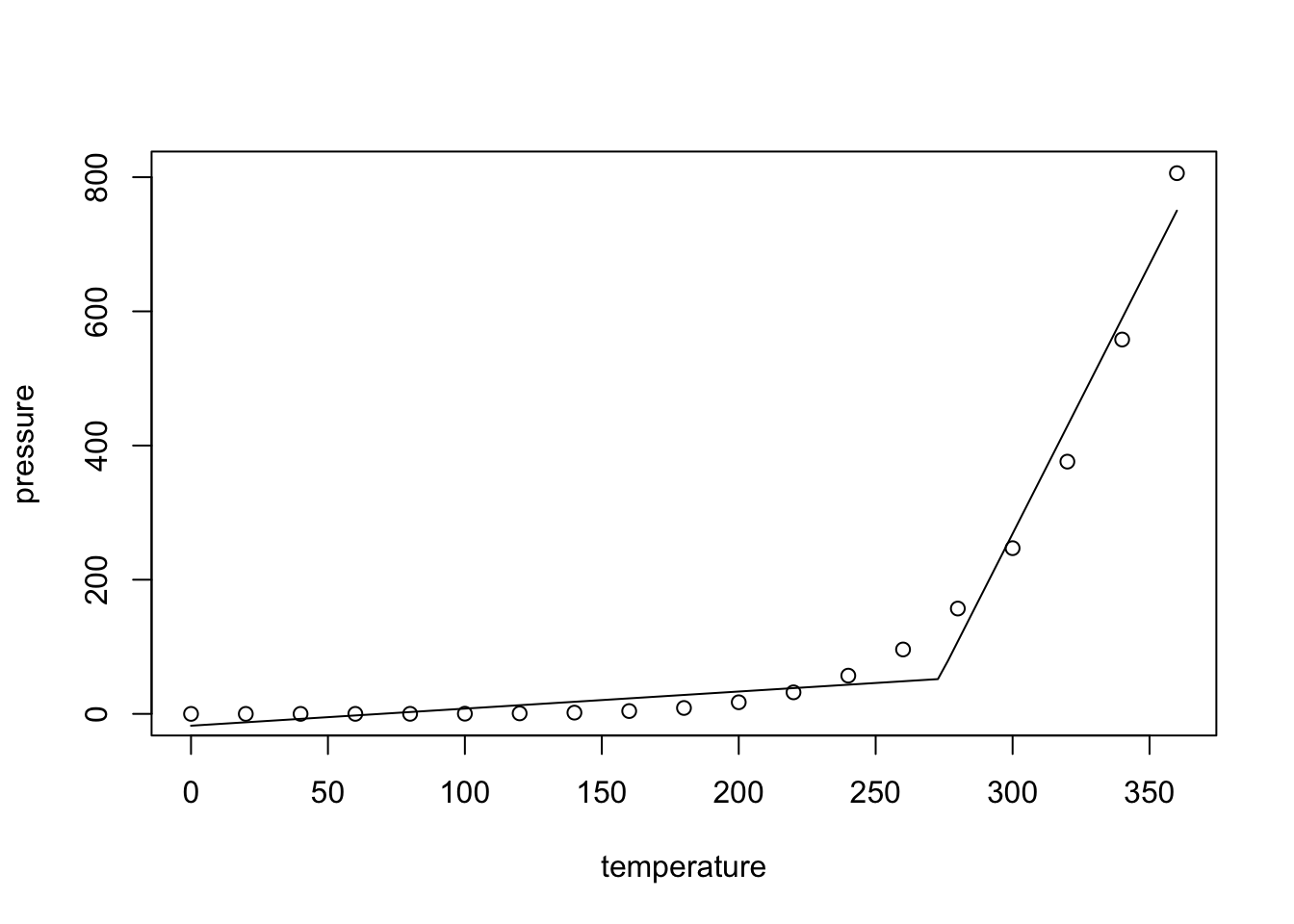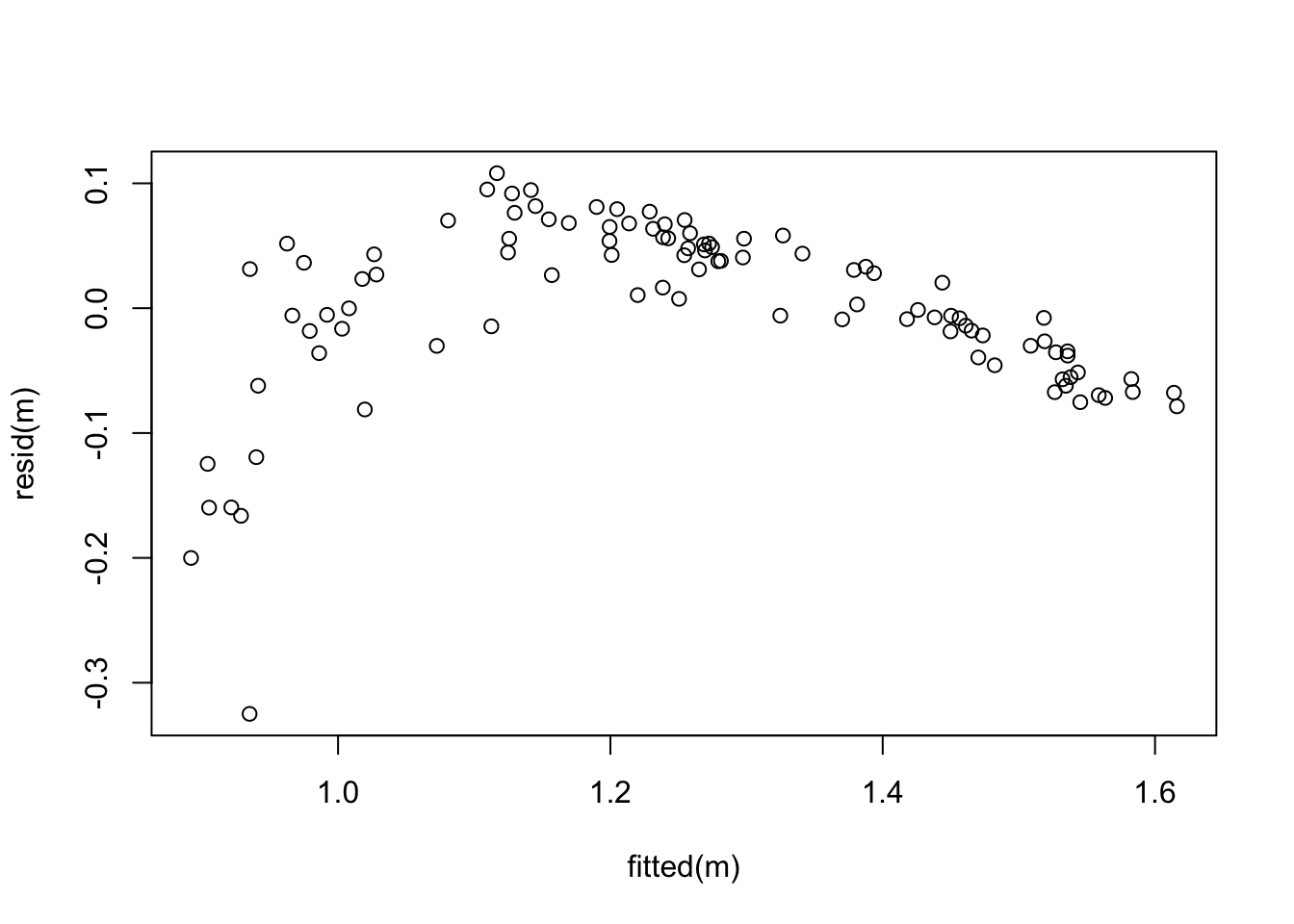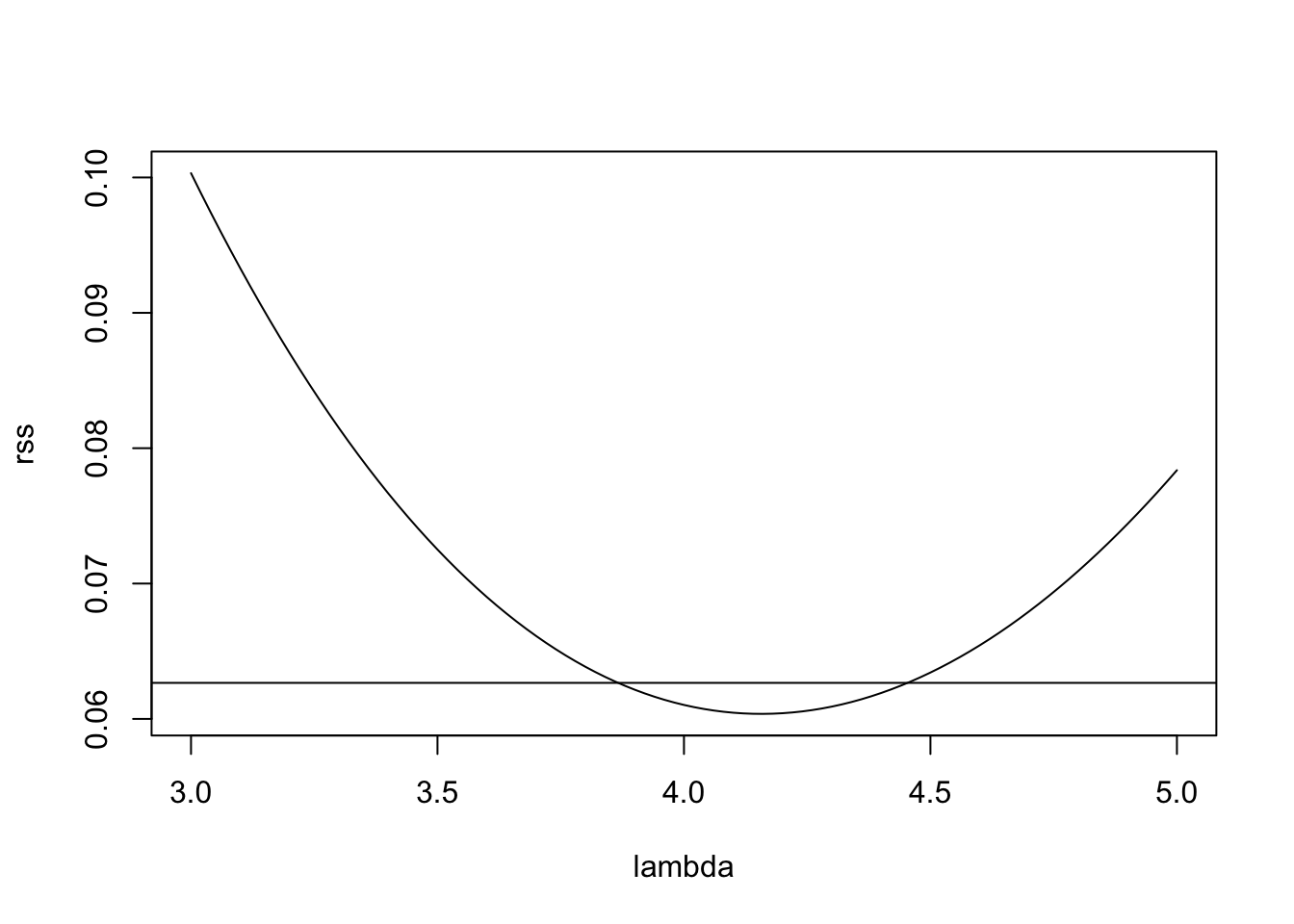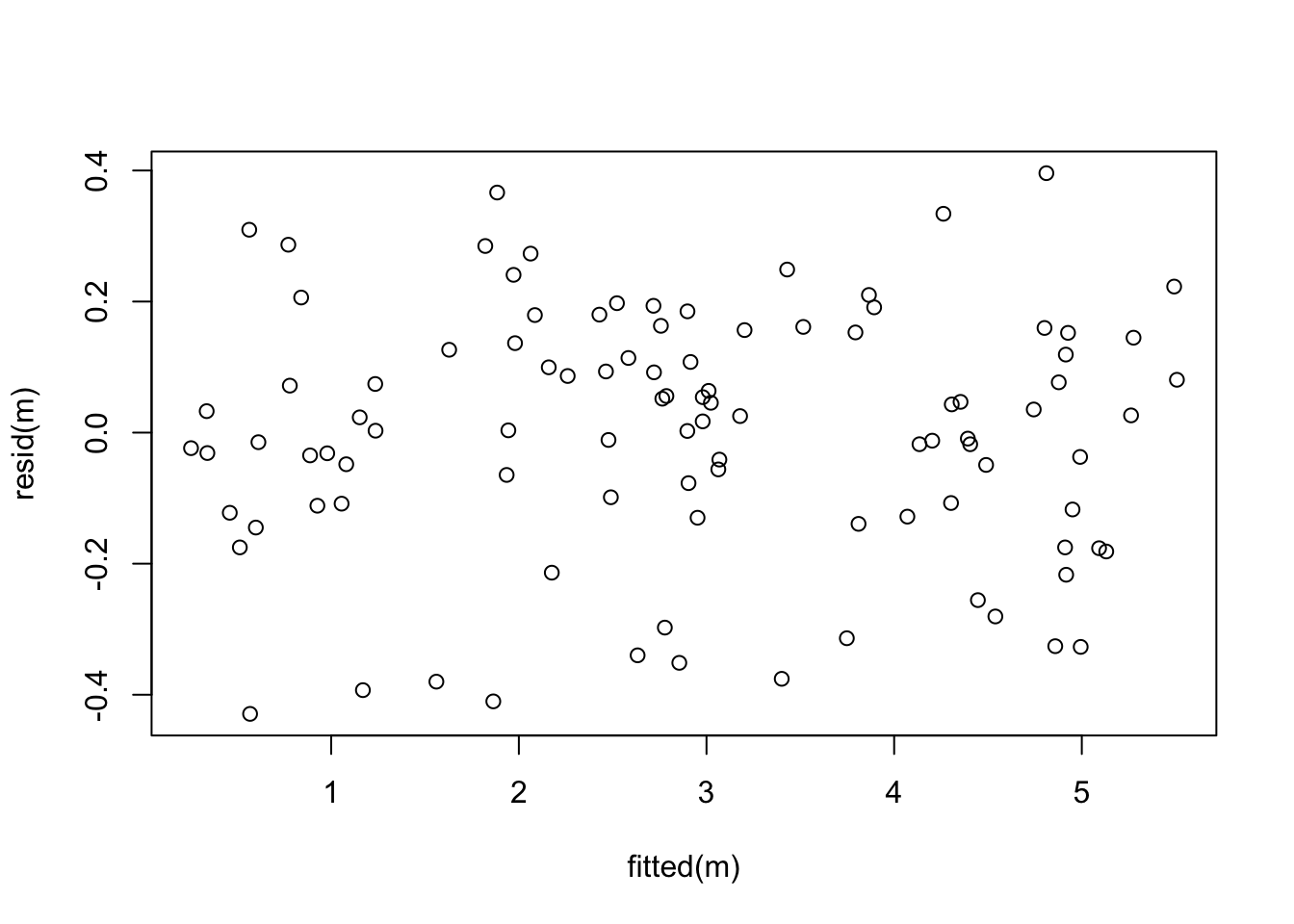# Problem Sheet 4

You should attempt all these questions and write up your solutions in advance of the workshop in week 8 where the answers will be discussed.

13. Consider the dataset pressure, built into R, which describes the vapour pressure $$P$$ of mercury as a function of temperature $$T$$.

1. For given $$T_0$$, explain how a model of the form $$P = \beta_0 + T \beta_1 + \max(0, T-T_0) \beta_2 + \varepsilon$$ can be fitted to the data.

We can fit a model with an intercept and two inputs, $$T$$ and $$\max(0, T-T_0)$$. In R we can use the following commands to fit such a model:

T0 <- the.given.value # substitute the value of T0 here
m <- lm(pressure ~ temperature + I(pmax(0, temperature-T0)),
data = pressure)
1. Why might a model of this form make sense for the given data?

Such a model may make sense, because the slope of the samples increases faster than linear as the temperature $$T$$ increases. The term $$\max(0, T-T_0)$$ is zero for small $$T$$, but adds an additional slope once $$T$$ exceeds the cutoff temperature $$T_0$$.

1. What is a good value of $$T_0$$ for this dataset?

$$T_0$$ should be placed at the end of the “flat” part of the plot, where the pressure starts increasing more quickly. A quick experiment shows that the best $$R^2$$ value is achieved for $$T_0 \approx 273$$:

T0 <- 273
m <- lm(pressure ~ temperature + I(pmax(0, temperature - T0)),
data=pressure)
summary(m)

Call:
lm(formula = pressure ~ temperature + I(pmax(0, temperature -
T0)), data = pressure)

Residuals:
Min      1Q  Median      3Q     Max
-53.125 -17.553  -6.308  13.017  55.839

Coefficients:
Estimate Std. Error t value Pr(>|t|)
(Intercept)                  -17.62933   14.63735  -1.204   0.2459
temperature                    0.25472    0.08774   2.903   0.0104 *
I(pmax(0, temperature - T0))   7.77118    0.38024  20.437 6.85e-13 ***
---
Signif. codes:  0 '***' 0.001 '**' 0.01 '*' 0.05 '.' 0.1 ' ' 1

Residual standard error: 29.86 on 16 degrees of freedom
Multiple R-squared:  0.9843,    Adjusted R-squared:  0.9823
F-statistic: 501.3 on 2 and 16 DF,  p-value: 3.705e-15

To get a better understanding of how the extra term $$\max(0, T-T_0)$$ improves model fit, we plot the data together with the fitted model:

plot(pressure)
T.plot <- seq(0, 360, l=100)
p.plot <- predict(m, data.frame(temperature=T.plot))
lines(T.plot, p.plot)As expected, for $$T > 273 = T_0$$, the slope of the fitted line increases, leading to greatly improved model fit. (An alternative, and probably superior approach would be to transform the data and try to fit a straight line to the transformed data instead.)

14. Where possible, rewrite the following regression models in linear form.

1. $$y = \beta_0 \beta_1^x$$

We can take logarithms: $\begin{equation*} \log(y) = \log(\beta_0) + x \log(\beta_1) =: \beta_0' + x \beta_1'. \end{equation*}$ Notice that writing $$\log(y) = \beta_0' + x \beta_1' + \varepsilon$$ implies $$y = \beta_0 \beta_1^x \exp(\varepsilon)$$.

1. $$y = \beta_0 + \beta_1 \sin(x_1) + \beta_2 \exp(x_2)$$

We can simply set $$x_1' = \sin(x_1)$$ and $$x_2' = \exp(x_2)$$. This transformation does not affect the error term.

1. $$y = \frac{x}{\beta_0 + \beta_1 x}$$

We can write $\begin{equation*} \frac1y = \frac{\beta_0 + \beta_1 x}{x} = \beta_0 \frac1x + \beta_1. \end{equation*}$ Thus we can use $$x' = 1/x$$, $$y' = 1/y$$, $$\beta_0' = \beta_1$$ and $$\beta_1' = \beta_0$$. This transformation affects the noise, too, so some care is needed.

15. Consider the dataset from

The dataset contains samples $$(x_{i,1}, x_{i,2}, y_i)$$. We want to find a model for $$y$$ as a function of $$x_1$$ and $$x_2$$.

1. Fit a linear model to the data and produce a residual plot. Based on the plot, discuss how well the model fits the data.

We can fit the model as follows:

# data from https://www1.maths.leeds.ac.uk/~voss/2022/MATH3714/P04Q14.csv
m <- lm(y ~ x1 + x2, data = d)
plot(fitted(m), resid(m))The mean of the residuals does not form a straight line, so the dependency of $$y$$ on the $$x_i$$ seems to be nonlinear. Also, the variance of the residuals decreases as $$\hat y$$ increases. Model fit is not good and a transformation of the data may be useful.

1. Apply a Power Transform to the model, as described in section 11.3 of the notes. Explain your choice of $$\lambda$$.

To choose the exponent $$\lambda$$, we plot the residual sum of squares as a function of $$\lambda$$.

gm <- exp(mean(log(d$y))) lambda <- seq(3, 5, length.out = 101) rss <- numeric(length(lambda)) for (i in seq_along(lambda)) { li <- lambda[i] y.prime <- (d$y^li - 1) / (li * gm^(li-1))
mi <- lm(y.prime ~ x1 + x2, data = d)
}

cutoff <- min(rss) * (1 + qt(0.971, 51)^2 / 51)
abline(h = cutoff)The horizonal line in this plot indicates the cutoff suggested by the rule of thumb in (11.1). Of the values of $$\lambda$$ where rss is below the cutoff, $$\lambda = 4$$ seems the most “simple”, so we try this value here.

y.prime <- d\$y ^ 4
m <- lm(y.prime ~ x1 + x2, data = d)
plot(fitted(m), resid(m))Now the residual plot looks perfect!

16. The Prediction Error Sum of Squares (PRESS) is defined as $\begin{equation*} \mathrm{PRESS} = \sum_{i=1}^n \bigl( y_i - \hat y^{(i)}_i \bigr)^2. \end{equation*}$ Using lemma 13.1, or otherwise, determine the PRESS value for the stackloss dataset built into R (using stack.loss as the response variable).

We can use the formula $\begin{equation*} \mathrm{PRESS} = \sum_{i=1}^n \Bigl( \frac{\hat\varepsilon_i}{1 - h_{ii}} \Bigr)^2 \end{equation*}$ from the lemma. Implementation in R is straightforward.

m <- lm(stack.loss ~ ., data = stackloss)
X <- model.matrix(m)
H <- X %*% solve(t(X) %*% X, t(X))
hii <- diag(H)
sum((resid(m)/(1 - hii))^2)
 291.8689